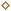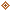;".date("d/m/Y")."\n"); fclose (\$fp); \$status="\$em has been removed."; unset(\$em); } else if(\$product=="activesmart" || \$activesmart=="on") { \$file="asmart-list.csv"; \$listname="Active SMART announcements mailing list"; } else if(\$ntpager=="on") { \$file="ntpager-list.csv"; \$listname="Ariolic NTPager announcements mailing list"; } else \$file="list.csv"; \$email=trim(strtolower(\$subscr_email)); \$username=\$subscr_name; \$is_deleted=false; function test_mail(\$char) { if(eregi("^[_\.0-9a-z-]+@([0-9a-z][-0-9a-z\.]+)\.([a-z]{2,3}\$)",\$char)) return true; return false; } function check_dupe(\$email) { global \$file; \$f_email=0; // field number of Email in CSV file \$f_username=1; // field number of Username in CSV file \$found_dupe=false; \$fp = fopen (\$file,"r"); while(\$data = fgetcsv (\$fp, 1000, ",")) { if(\$data[\$f_email]==\$email) { \$found_dupe=true; break; } } fclose (\$fp); return \$found_dupe; } if(count(\$HTTP_POST_VARS)>1) { if(!empty(\$email)) { if(test_mail(\$email)) { if(!empty(\$product)) { if(\$subscribe=="add") { if(!check_dupe(\$email)) { // add user to the list \$fp = fopen (\$file,"a+"); fputs(\$fp,"\$username;<\$email>;".date("d/m/Y")."\n"); fclose (\$fp); \$status="\$email added to \$listname"; unset(\$subscr_name); unset(\$subscr_email); } else \$status="The email address \$email already exists in \$listname"; } else if(\$subscribe=="remove") { // remove user from the list \$fcontents = file(\$file); for(\$i=0;\$i Ariolic Software - NewsletterAbout UsPress RoomClientsNewsletterContact Us